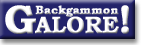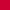Probability and StatisticsChance of rolling x doubles in y rolls

 From: Raccoon Date: 15 July 2007 Subject: Chance of rolling X doublets in Y rolls Forum: GammOnLine

```Chance of rolling exactly X doublets in Y rolls =

Y!      5^(Y-X)
--------- * -------
X!*(Y-X)!     6^Y

Example: chance of rolling exactly 1 doublet in 3 rolls =

3!      5^(3-1)   3*2   5^2    75
--------- * ------- = --- * --- = --- = 34.72%
1!*(3-1)!     6^3      2    6^3   216

Example: chance of rolling exactly 2 doublets in 4 rolls =

5!      5^(4-2)    4*3*2*1    5^2   24    25     150
--------- * ------- = --------- * --- = -- * ---- = ---- = 11.57%
2!*(4-2)!     6^4     2*1 * 2*1   6^4    4   1296   1296

Example: chance of rolling 8 doubles in 12 rolls simplifies to

495 * 5^4th * 6^12th = 0.0142%

Example: if X = O, then the chance of rolling 0 doubles in Y rolls is
simply 5^Y/6^Y.
```

 Douglas Zare  writes: ```> What if the question is x OR MORE doubles in y rolls? The simplest method (with a computer) is to add up the possibilities. That's what I usually do. A good(*) approximation when the number of rolls is large is to translate the result of "x or more doubles" into "z or more standard deviations above the mean," and then use a normal approximation, looking up the value z in a table. The mean is y/6. The standard deviation is sqrt(y * 5/36) or about sqrt(y) *3/8. x is x-(y/6) above the mean, which is x-(y/6) / sqrt(y * 5/36) above the mean. However, the number of doubles is a count, but the normal distribution is continuous, so you should replace "x" with "from x-1/2 to x+1/2," and "x or more" by "x-1/2 or more." So, "x or more" should be translated to (x-1/2 - y/6)/sqrt(y * 5/36) standard deviations above the mean. For example, lets look at the statement Kit Woolsey made a while back that 200 or more doubles in 1000 rolls would seem suspicious. That is 199.5 - 166.7 / sqrt(5000/36) = 32.8 / 11.8 ~ 2.79 standard deviations above the mean, which happens about 1 time in 375. Good intuition, Kit. The actual value is about 1/314. * For extreme values, the absolute error may be small, while the proportional error is large. For example, you will get a small positive estimate for the probability of at least y+1 doubles in y rolls, when the actual probability is 0. Unfortunately, we are often interested in such cases where the probability is small, such as in the above example. > What are the odds (%) that one will roll x# doubles more than his > opponent? If it were a fixed number of rolls, you can just add up the possibilities, or use a normal approximation to each, and then the difference between normally distributed random variables is also normally distributed. If it is from a backgammon game, then it depends greatly on the styles of the players, since it is much easier to roll more doubles when the game is longer, or in live play, when your opponent doesn't roll for a long time. Douglas Zare ```

### Probability and Statistics

Average game and match length  (JP White, Dec 2000)
Average luck of each roll  (Timothy Chow+, Mar 2013)
Average luck of each roll  (Jørn Thyssen+, Feb 2004)Calculating winning chances  (Douglas Zare, June 2000)
Chance of rolling x doubles in y rolls  (Raccoon+, July 2007)Chance of rolling x or more pips in y rolls  (Tom Keith, Feb 2004)Clumping of random numbers  (Gary Wong, Sept 1998)
Counting shots  (Koyunbaba+, June 2007)
Counting shots  (John Little+, Mar 2007)Distribution of points per game  (Roland Sutter, June 1999)
Distribution of points per game  (Stig Eide+, Sept 1995)Expected variation in points after a series of games  (Achim Müller+, Feb 1999)
How many games to decide who's better?  (Stephen Turner, Mar 1997)
How often is too often?  (Gary Wong, Oct 1998)
Losing after bearing off 14 checkers  (Daniel Murphy, July 1999)
Number of games per match  (Jason Lee+, Jan 2005)
Number of rolls to enter x checkers from bar  (Michael Depreli+, Mar 2011)
Visualizing odds  (Daithi, Mar 2011)From GammOnLineLong messageRecommended readingRecent addition

 Book Suggestions Books Cheating Chouettes Computer Dice Cube Handling Cube Handling in Races Equipment Etiquette Extreme Gammon Fun and frustration GNU Backgammon History Jellyfish Learning Luck versus Skill Magazines & E-zines Match Archives Match Equities Match Play Match Play at 2-away/2-away Miscellaneous Opening Rolls Pip Counting Play Sites Probability and Statistics Programming Propositions Puzzles Ratings Rollouts Rules Rulings Snowie Software Source Code Strategy--Backgames Strategy--Bearing Off Strategy--Checker play Terminology Theory Tournaments Uncategorized Variations